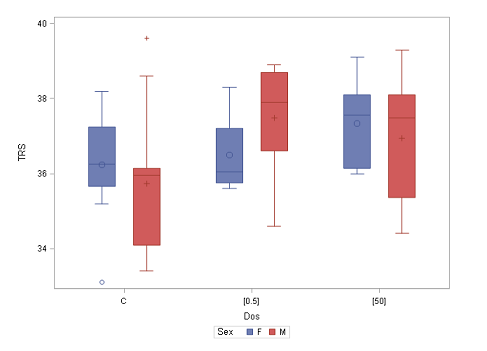28
Feb

1
Apr
Umeå

# Statistics I: Basic statistics, 4 hpThe Ph.D. course will be given in Umeå 28 February - 1 April 2022.

#### Objectives

The objective of the course is to give an introduction to basic probability theory and statistical inference. On completion of the course, the student will be able to:
• use basic probability concepts and statistical principles
• formulate a problem in statistical terms
• describe common statistical methods including conditions and assumptions
• apply basic statistical methods in scientific research
• select an appropriate statistical method for a given problem
• carry out a basic statistical analysis
• interpret and evaluate results correctly and draw reasonable conclusions
• clearly and concisely communicate results and conclusions
• use statistical software for analysis

#### Content

The course is built on lectures and computer exercises. The main moments are as follows:
• Descriptive statistics.
• Basic probability theory.
• Probability distributions.
• Statistical inference of means and proportions, one and two samples.
• Nonparametric and resampling methods.
• Analysis of frequency data.
• Sample size determination.

## Facts

Time: 2022-02-28 - 2022-04-01
City: Umeå
Last signup date: 7 February 2022